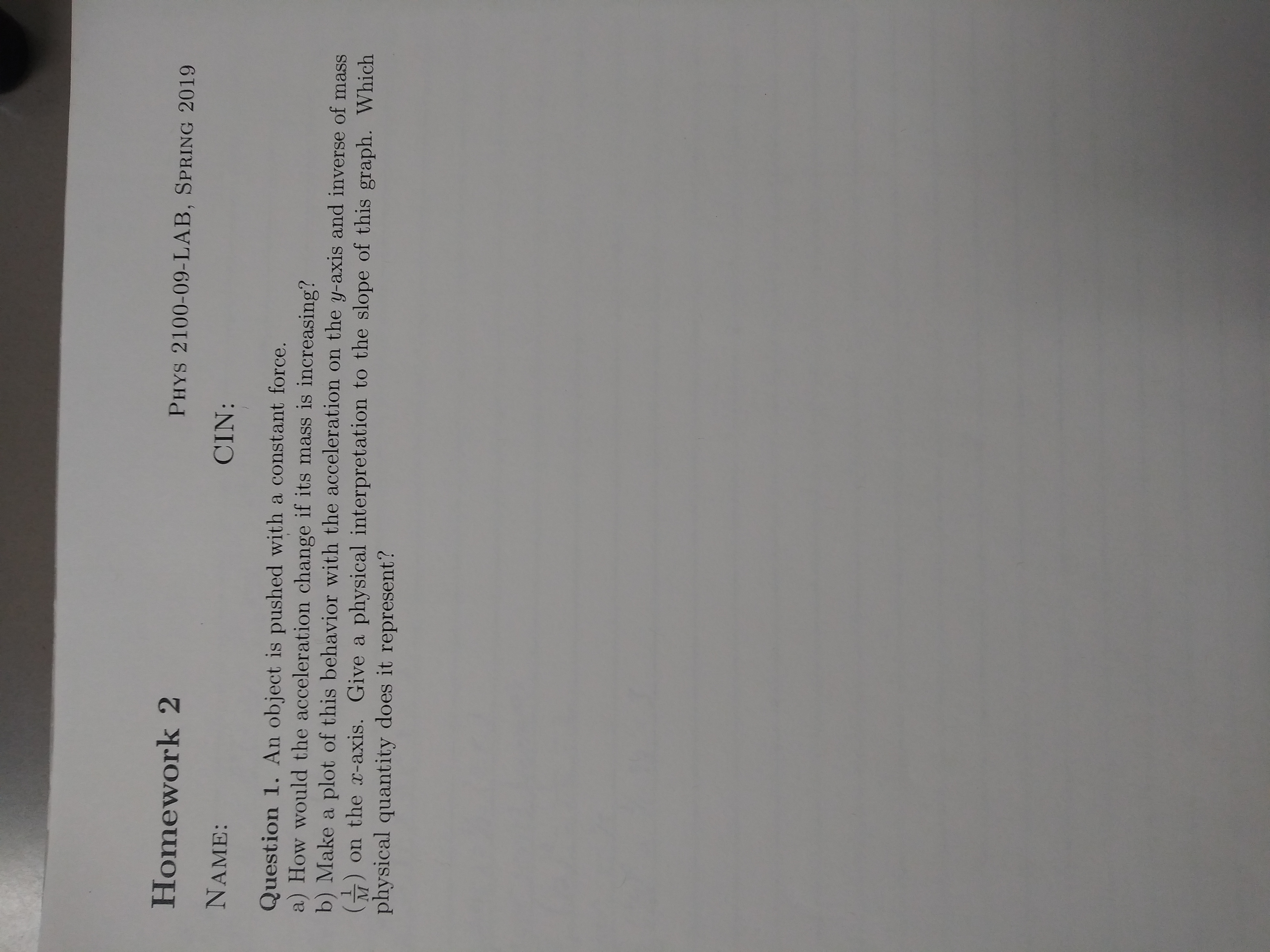# Homework 2NAME:Question 1. An object is pushed with a constant force.PHYS 2100-09-LAB, SPRING 2019CIN:a) How would the acceleration change if its mass is increasing?b) Make a plot of this behavior with the acceleration on the y-axis and inverse of mass) on the x-axis. Give a physical interpretation to the slope of this graph whichphysical quantity does it represent?

Question
48 viewshelp_outlineImage TranscriptioncloseHomework 2 NAME: Question 1. An object is pushed with a constant force. PHYS 2100-09-LAB, SPRING 2019 CIN: a) How would the acceleration change if its mass is increasing? b) Make a plot of this behavior with the acceleration on the y-axis and inverse of mass ) on the x-axis. Give a physical interpretation to the slope of this graph which physical quantity does it represent? fullscreen
check_circle

Step 1

a)

The relation of force, mass and acceleration is given by the Newton’s second law.

Step 2

When force is constant, the mass and acceleration are inversely proportional to each other.

Step 3

Ans: when the force is constant and the mass is i...

### Want to see the full answer?

See Solution

#### Want to see this answer and more?

Solutions are written by subject experts who are available 24/7. Questions are typically answered within 1 hour.*

See Solution
*Response times may vary by subject and question.
Tagged in

### Newtons Laws of Motion